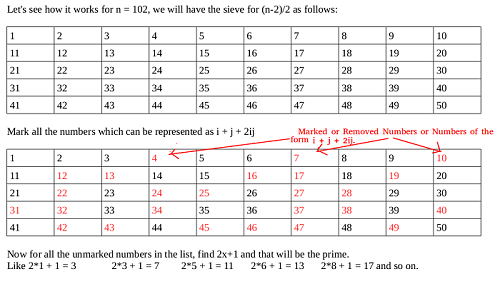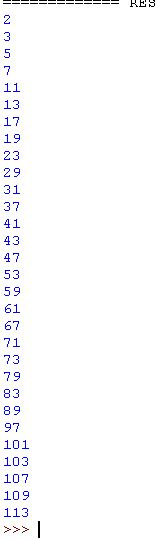×

Search anything:

# Find all primes with Sieve of Sundaram

#### Algorithms List of Mathematical Algorithms prime numberSieve of Sundaram is an efficient algorithm used to find all the prime numbers till a specific number say N. This algorithm was discovered by Indian mathematician S. P. Sundaram in 1934. It performs better than popular methods like Sieve of Eratosthenes for smaller values till 5000.

There are different approches to do this task :

1. Naive approach
2. Improved version of naive approach
3. Sieve of Eratosthenes
4. Sieve of Atkin

We will take a brief look into the common and simple approaches to this problem before going into the step by step explanation of Sieve of Sundaram

## Naive approach

The key idea is to check if a given number N is a prime of not by dividing it by all numbers less than N. So, to find all prime numbers upto N, it takes O(N^2) time complexity.

Pseudocode:

``````prime_set = {2}
for(int i=3; i<N; i++)
{
boolean check = true;
for(int j=2; j<i; j++)
if(i%j == 0)
{
check = false;
break;
}
if (check)
}
``````

This approach can be improved by checking only for numbers till square root of N for a given number N. It will reduce the time complexity to O(N^1.5)

## Sieve of Eratosthenes

The key idea in Sieve of Eratosthenes is to check a number by dividing it by only primes that appear before this. As the density of primes is significantly less, this gives a massive boost.

Time Complexity of Sieve of Eratosthenes is: O(N * log(log(N))))

Learn about Sieve of Eratosthenes in depth

Time complexity with sieve of Atkin goes down to O(N)

Learn about Sieve of Atkin in depth

# Approach of Sieve of Sundaram

Let us say we need to find the prime numbers till 15 (n=16)

• Step1: We find the (integer) value of n-2/2 and let it be in k
• Step2: Now fill the list with the numbers 1 to k
• Step3: Now remove the numbers that are of the form i+j+2ij where 1<=i<=j,i+j+2ij<=k

For Example if we see the case below for number 120,

first i=1 and j=1 and the value of i+j+2ij =4 and hence 4 is removed which is needed as well as we know 4 is not a prime number (also on observation we can check that 2x+1 ie 2 * 4+1=9 which is also not a prime number so for 4 there is no way to get prime number) .This is how the process continues for i and j as given above.

• Step4: Now for left numbers 2*x+1 (x is the number in the list) gives the prime number.

Reason for Time complexity:

Time taken to iterate over N numbers and mark non-primes, T(N)=N+T(N−1)

So time taken in next step (N - 1) will be, T(N−1)=N/2+T(N−2)

To double the rest and increment by one, it will constant time for each. Total of O(N) which is definitely less than the time taken for removing non-primes.
Therefore, T(N)=N+N/2+N/3+…+1=O(NlogN)

Pictorial Explanation## Step by step Explanation

step1: Let n=20
step2: k=20-2/2=9
step3: Let's understand by table given below

 1 2 3 4 5 6 7 8 9

step4: for 1<=i<=j thus , case when i=1 and j=1,the value of i+j+2ij=4<=9(True) thus we remove 4

 1 2 3 4 5 6 7 8 9

step5: now for i=1 and j=2 i+j+2ij=7<=9 thus we remove 7

 1 2 3 4 5 6 7 8 9

step6: now for i=1 and j=3 i+j+2ij=10>9 thus break

 1 2 3 4 5 6 7 8 9

step7: now i=i+1 ,i=2 and j=2 i+j+2ij=12>9 thus break as similarly i=3 and j=3 gives 25>9 and so on ...

 1 2 3 4 5 6 7 8 9

step8: so in total numbers left are 1,2,3,5,6,8,9

 1 2 3 4 5 6 7 8 9

step9: Hence now we calculate values 2*x+1 for these values to get all primes nos upto n (along with 2)

step10: The coloured numbers are x values for 2*x+1 as below (including 2):
2*1+1=3
2*2+1=5
2*3+1=7
2*5+1=11
2*6+1=13
2*8+1=17
2*9+1=19
Hence got all prime number till 20.

 1 2 3 4 5 6 7 8 9

### Implementation in Python

``````def Sieveofsundaram(n):
k = int((n - 2) / 2)
a =  * (k + 1)
for i in range(1, k + 1):
j = i
while((i + j + 2 * i * j) <= k):
a[i + j + 2 * i * j] = 1
j += 1
if (n > 2):
print(2," ")
for i in range(1, k + 1):
if (a[i] == 0):
print((2 * i + 1)," ");
n=120
Sieveofsundaram(n)
``````

## Implementation in C++

``````#include <bits/stdc++.h>
using namespace std;
int SieveOfSundaram(int n)
{
int k = (n-2)/2;
bool a[k + 1];
memset(a, false, sizeof(a));
for (int i=1; i<=k; i++)
for (int j=i; (i + j + 2*i*j) <= k; j++)
a[i + j + 2*i*j] = true;

if (n > 2)
cout << 2 << " ";

for (int i=1; i<=k; i++)
if (a[i] == false)
cout << 2*i + 1 << " ";
}

int main(void)
{
int n = 120;
SieveOfSundaram(n);
return 0;
}
``````

Result:## Comparison between Sieve of Eratosthenes and Sieve of Sundaram

• For small numbers Sieve of Sundaram is better than Sieve of Eratosthenes ,
• For large numbers Sieve of Eratosthenes is better.

List of Numbers

 No Number Sieve of Eratosthenes Sieve of Sundaram 1 10 0:0:0.168 0:0:0.083 2 100 0:0:0.056 0:0:0.004 3 1000 0:0:0.542 0:0:0.188 4 5000 0:0:3.986 0:0:2.927 5 100000 0:0:25.983 0:0:27.349

### Time Comlexity

• Worst case time complexity: `Θ(nlogn)`
• Average case time complexity: `Θ(nlogn)`
• Best case time complexity: `Θ(n)`
• Space complexity: `Θ(n)`

## Question

#### Which one of the following is correct?

Sieve of sundaram is preferred for small numbers.
Sieve of Sundaram gives all prime numbers till n.
Time complexity of Seive of sundaram is O(logn)
Sieve of sundaram is better than sieve of atkin.
seive of sundaram is considered better for small numbers.(Time complexity is O(n*logn)) hence for large value of n O(n*logn) does not provide proficiency.)Also explained below.